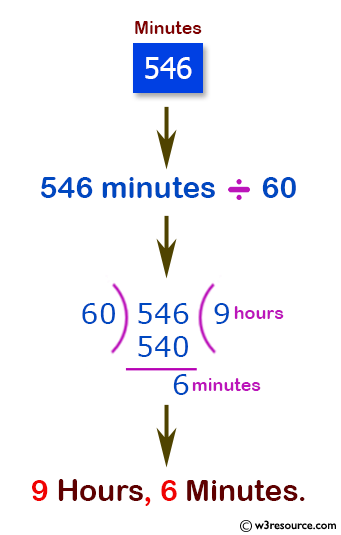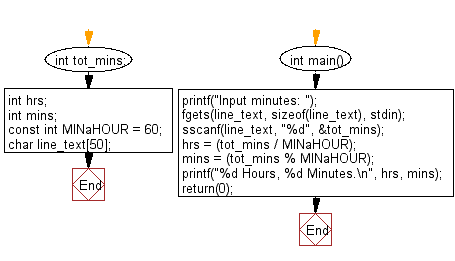﻿ C Program: Calculate the total number of hours and minutes - w3resource# C Exercises: Calculate the total number of hours and minutes

## C Input Output statement and Expressions: Exercise-6 with Solution

Write a program in C that takes minutes as input, and display the total number of hours and minutes.

Pictorial Presentation:Sample Solution:

C Code:

``````#include <stdio.h>
int tot_mins;  /* given number of minutes */
int hrs;          /* number of hours (to be computed) */
int mins;        /* number of minutes (to be computed) */

const int MINaHOUR = 60;      /* number of minutes in an hour */

char line_text;      /* line of input from keyboard */

int main() {
printf("Input minutes: ");
fgets(line_text, sizeof(line_text), stdin);
sscanf(line_text, "%d", &tot_mins);

hrs = (tot_mins / MINaHOUR);
mins = (tot_mins % MINaHOUR);

printf("%d Hours, %d Minutes.\n", hrs, mins);

return(0);
}
```
```

Sample Output:

```Input minutes: 546
9 Hours, 6 Minutes.
```

Flowchart:C Programming Code Editor:

Improve this sample solution and post your code through Disqus.

What is the difficulty level of this exercise?

Test your Programming skills with w3resource's quiz.

﻿